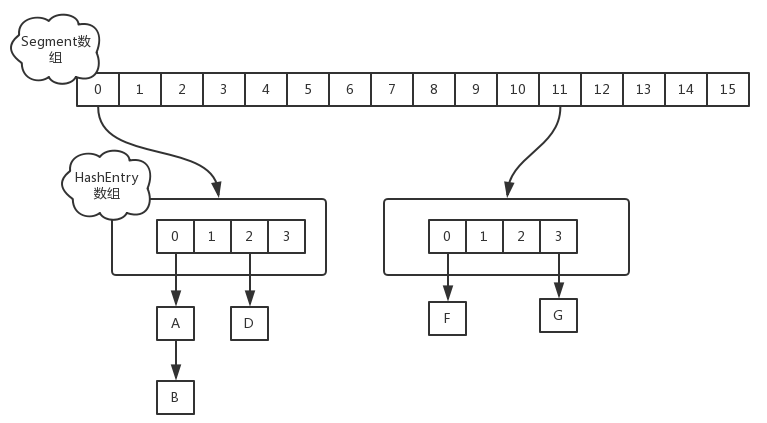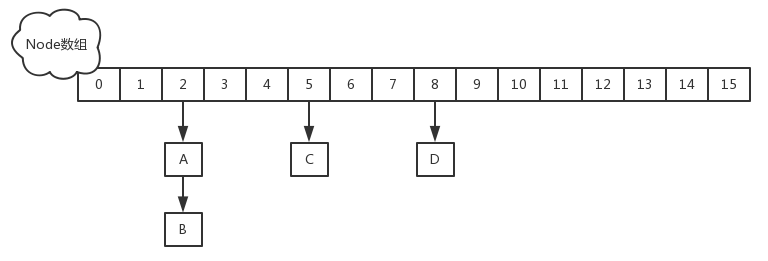# ConcurrentHashMap的高并发和线程安全

Posted by RussXia on May 9, 2019

# 为什么需要ConcurrentHashMap

HashMap并不是并发容器，在并发环境必定存在性能和安全等方面问题。HashMap对应的线程安全容器HashTable或者`java.util.Collections#synchronizedMap`返回的线程安全容器，归根结底都只是用`synchronized`修饰获得的线程安全，性能并不高。

# JDK1.7和1.8中ConcurrentHashMap的数据结构变化

## JDK 1.7中的ConcurrentHashMap

ConcurrentHashMap在1.7中的扩容方式仍然是基于2的幂数倍扩容(方便复制转移元素)。但是ConcurrentHashMap的扩容是针对某个Segment的，而不是针对整个容器的。

``````static final class Segment<K,V> extends ReentrantLock implements Serializable {
transient volatile int count;
transient int modCount;
transient int threshold;
transient volatile HashEntry<K,V>[] table;
}
``````
``````static final class HashEntry<K,V> {
final K key;
final int hash;
volatile V value;
final HashEntry<K,V> next;
}
``````## JDK 1.8中的ConcurrentHashMap

JDK1.8中的ConcurrentHashMap摒弃了分段锁的设计。在数据结构上采用数组+链表+红黑树的结构，和HashMap使用的数据结构是一样的。在线程安全方面，JDK1.8中的ConcurrentHashMap采用了 CAS + synchronized的设计，所以看起来ConcurrentHashMap看起来就像是一个优化版的线程安全的HashMap。

``````static class Node<K,V> implements Map.Entry<K,V> {
final int hash;
final K key;
volatile V val;
volatile Node<K,V> next;
}
``````# JDK1.8中的ConcurrentHashMap中如何保证线程安全

``````static final int MOVED     = -1; // hash for forwarding nodes
static final int TREEBIN   = -2; // hash for roots of trees
static final int RESERVED  = -3; // hash for transient reservations
static final int HASH_BITS = 0x7fffffff; // usable bits of normal node hash
``````
``````final V putVal(K key, V value, boolean onlyIfAbsent) {
if (key == null || value == null) throw new NullPointerException();
//计算当前key的hash值
int binCount = 0;
//如果这次没有put成功，会重试
for (Node<K,V>[] tab = table;;) {
Node<K,V> f; int n, i, fh;
//ConcurrentHashMap的初次put会做init操作
if (tab == null || (n = tab.length) == 0)
tab = initTable();
//如果对应的node为空，以cas的方式put元素，如果成功，直接退出循环
else if ((f = tabAt(tab, i = (n - 1) & hash)) == null) {
if (casTabAt(tab, i, null,
new Node<K,V>(hash, key, value, null)))
break;                   // no lock when adding to empty bin
}
//如果tab[i]的hash值为MOVED，表明该链表正在进行transfer操作,当前线程先帮助进行扩容操作,然后put
else if ((fh = f.hash) == MOVED)
tab = helpTransfer(tab, f);
else {
V oldVal = null;
//锁住node节点，对节点下的链表/红黑树进行同步操作
synchronized (f) {
if (tabAt(tab, i) == f) {
if (fh >= 0) {  //大于0说明当前node是一个可用的链表节点
binCount = 1;
for (Node<K,V> e = f;; ++binCount) {
K ek;
//如果当前key已经存在，判断是否新值替换旧值(根据传入的onlyIfAbsen决定),然后退出
if (e.hash == hash &&
((ek = e.key) == key ||
(ek != null && key.equals(ek)))) {
oldVal = e.val;
if (!onlyIfAbsent)
e.val = value;
break;
}
//如果下一个节点为空，直接put进去，然后退出
Node<K,V> pred = e;
if ((e = e.next) == null) {
pred.next = new Node<K,V>(hash, key,
value, null);
break;
}
//继续遍历
}
}
//如果当前节点是一个树状结构，向树中插入一个元素
else if (f instanceof TreeBin) {
Node<K,V> p;
binCount = 2;
if ((p = ((TreeBin<K,V>)f).putTreeVal(hash, key,
value)) != null) {
oldVal = p.val;
if (!onlyIfAbsent)
p.val = value;
}
}
}
}
//binCount是用来统计链表长度的
if (binCount != 0) {
//如果长度达到阈值，转化为红黑树
if (binCount >= TREEIFY_THRESHOLD)
treeifyBin(tab, i);
if (oldVal != null)
return oldVal;
break;
}
}
}
//统计长度，检查时候需要扩容